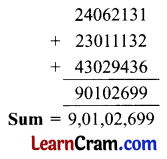# DAV Class 5 Maths Chapter 2 Worksheet 1 Solutions

The DAV Class 5 Maths Book Solutions Pdf and DAV Class 5 Maths Chapter 2 Worksheet 1 Solutions of Operations on Large Numbers offer comprehensive answers to textbook questions.

## DAV Class 5 Maths Ch 2 Worksheet 1 Solutions

Question 1.
Find the sum.
(а) 2,92,342; 14,54,651; 46,81,509
Solution:(b) 4,14,142; 49,85,389; 26,14,758
Solution: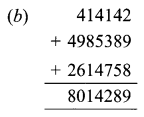(c) 3,00,286; 13,03,089; 85,09,10,008
Solution: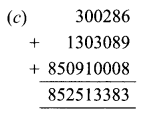(d) 60,32,85,862; 12,40,31,029; 7,01,96,253
Solution:(e) 1,82,95,067; 7,06,53,248; 85,23,15,901
Solution: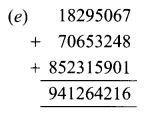(f) 8,43,26,198; 39,46,045; 1,83,49,730
Solution:(g) 1,23,45,678; 89,43,261; 5,97,86,009
Solution:(h) 4,02,36,754; 3,21,33,046; 2,95,17,354
Solution: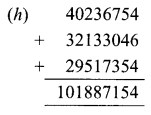Question 2.
Subtract.
(a) 13,91,803 from 52,09,123
Solution:(b) 25,18,624 from 40,00,000
Solution:(c) 3,65,17,298 from 8,79,25,149
Solution: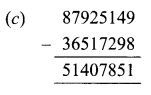(d) 73,82,005 from 90,28,583
Solution:(e) 3,62,71,843 from 4,98,07,916
Solution: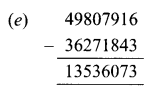(f) 3,89,07,392 from 8,13,00,898
Solution:(g) 6,23,94,389 from 8,03,09,421
Solution: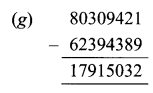(h) 1,98,76,432 from 5,23,45,678
Solution: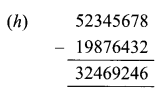Review Exercise

Question 1.
Find the sum.
(a) 4,38,291; 35,605 and 3,19,278
Solution: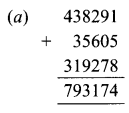(b) 95,262; 6,15,893 and 3,20,503
Solution: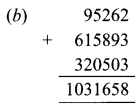Question 2.
Find the difference.
(a) 3,84,962 and 5,73,248
Solution: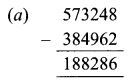(b) 9,00,000 and 7,11,498
Solution: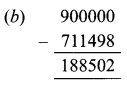Question 3.
Find the product.
(a) 4,908 by 326
Solution:(b) 11,321 by 74
Solution:Question 4.
(a) 4,182 by 13
Solution:Check:
Dividend = (Divisor × Quotient) + Remainder
R.H.S = (13 × 321) + 9
= 4173 + 9
= 4182
= L.H.S

(b) 2,000 by 45
Solution: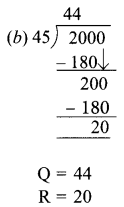Check:
Dividend = (Divisor × Quotient) + Remainder
R.H.S = (45 × 44) + 20
= 2000
= L.H.S

Question 5.
Ritesh is a kite maker. In one season, he sold 37,043 red kites, 42,620 blue kites, and 27,986 green kites. How many kites did he sell in all?
Solution:
Ritesh sold no. of red kites = 37043
Ritesh sold no. of blue kites = +42620
Ritesh sold no. of green kites = +27986
He sold a total of kites = 107649
Ritesh sold 1,07,649 kites in all.Question 6.
If a factory produces 1,285 toy cars every day, how many toy cars will it produce in a year of 293 working days?
Solution:
In 1 day factory produces toy cars = 1285
In 293 days factory will produce toy cars = 1285 × 293 = 3,76,505
It will produce 3,76,505, toy cars in 293 working days.

Question 7.
In a year, Rahul earns ₹ 72,600. How much will he earn monthly?
Solution:
1 year = 12 months.
In 12 months, Rahul earns = ₹ 72600
In 1 month, Rahul earns = ₹ 72600 ÷ 12 = ₹ 6,050
He will earn ₹ 6,050 monthly.

Question 8.
Fill in the blanks.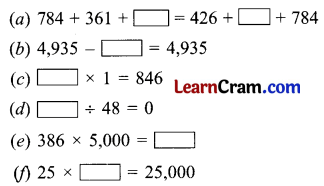Solution:DAV Class 5 Maths Chapter 2 Worksheet 1 Notes

• When some numbers are added, each number is called an addend.
• The sum of two numbers does not change when the order of addends is changed. This is called the order property of the addition of numbers.
• The sum of three or more numbers does not change when their grouping is changed.
• In the case of subtraction, The larger number is called minuend, The number to be subtracted is called subtrahend.
• We cannot change the order of numbers in subtraction.
• When two or more numbers are multiplied to give a product, then each of the numbers is called a factor of the product.
• Dividend = (Divisor × Quotient) + Remainder.Addition and Subtraction of Large Numbers
We have to add or subtract large numbers in the same way as we added and subtracted 5-digit and 6-digit numbers.

Example 1.